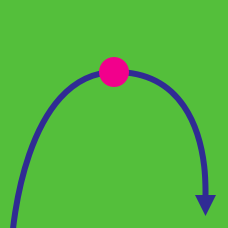Calculus

# Optimization Problems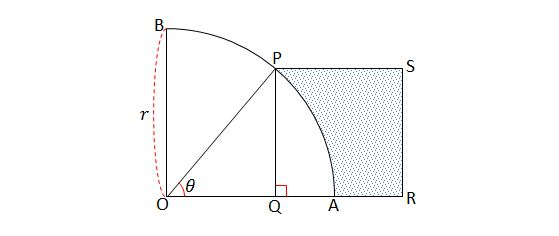In the above diagram, $P$ is on the arc $AB$ of a quarter of a circle $OAB$ with radius $r=13,$ and the line segment $\overline{PQ}$ is perpendicular to $\overline{OA}.$ If $\square PQRS$ is a square and the area of the shaded region is $T,$ what is the length of $\overline{PQ}$ that maximizes $T?$

A shop sells $500$ smartphones a week for $\450$ each. A market survey shows that each decrease of $\5$ on the price will result in the sale of an additional $10$ smartphones per week. What price of the smartphone would result in maximum revenue?

Details and Assumptions:

• The revenue is defined as the product of the number of items sold and the price of each item.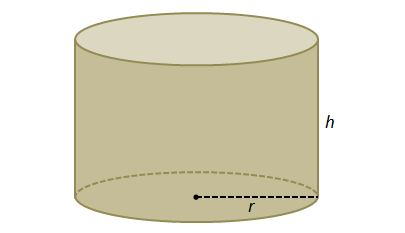A closed cylinder made of an iron plate can contain $3000 \text{ cm}^3$ of liquid. What is the radius of the cylinder (in cm) that minimizes the use of the iron plate? (Suppose the thickness of the iron plate is negligible.)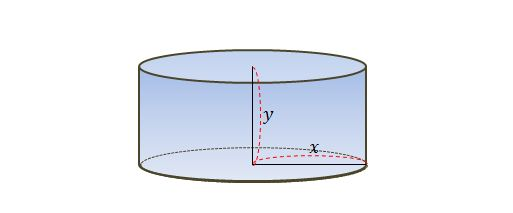If the sum of the radius and height of a cylinder is $45\text{ cm},$ what is the maximum volume of the cylinder?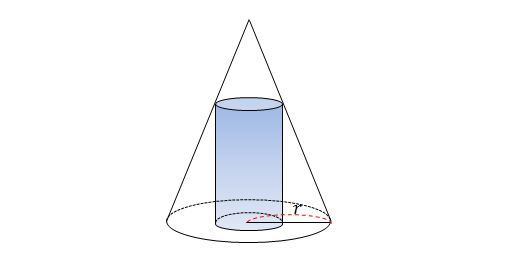As shown in the diagram above, a cylinder is inscribed in a right circular cone with base radius $r=60\text{ cm}.$ What is the radius of the cylinder (in cm) that maximizes the volume of the cylinder?

×